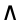# Errata -- First Revised Edition)

## (Second printing, March 2009)

You have a First Revised Edition of the book if the Morgan-Kauffmann logo is at the lower right corner of the cover. If not, check the Errata for the First Edition.

In the second printing of the First Edition, we have fixed all major first edition errata, and almost all of the minor errata. However, there were some non-erroneous additions that would have involved too much additional text and have led to impermissible page roll-overs. Here they are, together with any errata that may have been found after publication.

This is the bitmap symbol version of this page - not all browsers support all standard html symbols (older versions of Internet Explorer, for example). If you think that this symbol "" does NOT look like a square, you may want to view normal version.

Those errata in bold correspond to Major Corrections; those marked with '*' correspond to Minor Corrections, and those that are unmarked correspond to Typos.

• Pg 85, line 19,20: "dual by B" is perhaps too colloquial, since dualization always involves the inverse B-1; so "dual by B-1" would be more accurate. [20100828 AV]
• *Pg 113, line -3: Change also a linear transformation to also an outermorphism. 
• Pg 130, eq 5.10: Here we use the undualization of pg.81 for the first time. [20100830 AV]
• Pg 158, Figure 6.4: In view of the text, it would be more consistent to label the result as axa-1 [20100830 AV]
• Pg 160, a simpler example is grade 2 of the product of the blades e1e3 and (e1-e2)(e3-e4) . [20100201 AS]
• Pg 192, eq. 7.18: change x to X. [20120214 TC]
• *Pg 225, bottom derivation: AC reports a more compact derivation:
limε→0 ((xa)-1-x-1)/ε = limε→0 -x-1 ((xa)-x)/ε) (xa)-1 = -x-1 a x-1. [20071127 AC]
• **Pg 237, entry XX should not be m but 'm choose grade(X)' so (grade(X)m). 
• *Pg 286, section 11.5.3: This would be a good place to be more precise about the definition of weight. Write the direction A of a finite k-flat X in terms of a chosen pseudoscalar Ik for its subspace as A = ω Ik , then ω is the weight of the blade. For an infinite (k+1)-blade X, choose a pseudoscalar Ik+1 and write X = ω Ik+1, then ω is the weight of the blade. In both cases, the sign of the weight is determined by the chosen orientation of the pseudoscalar, but its magnitude is geometrically objective. It is simple to prove from the definition that a weight is translation invariant. Note also that unit weight and unit norm are different concepts; for the point representation e0+p has weight 1, but norm √(e02+p2) [20080326 AC]
• *Pg 296, figure 11.7: Because of the counter-intuitive signs resulting in (a) and (c), AC has the sensible suggestion to redefine the relative orientation of A to B as BA (so that the orientator is written first, the orientatee last). [20080326 AC]
• *Pg 389, structural exercise 9: There are only four degrees of freedom in this motion, so it is not the general RBM. Determine it anyway. 
• *Pg 407-409: We should have defined more clearly what we mean by weight and orientation as the split of the geometrical concept direction into a scalar and a unit blade element. In the caption of Table 14.2, weight is defined in a manner that makes it always positive -- but this then presumes that the orientation will pick up the sign. AC clarifies the issue raised in the second paragraph of page 409:
Let D = Ek ∞ be the direction of a conformal blade X.
If a unit orientation Ik has been given for the k-D vectorspace of Ek, and Ek = α Ik, then α is the weight of Ek, and the orientation of X is sign(α) Ik.
If k=0, the standard orientation is 1, and if k=n, the standard orientation is In. These are invariant under rigid body motions; for the other k-values the orientations are still invariant under translations.
[20071130 AC]
• Pg 476, line 2: replace two spheres by two equal-radius spheres. [20120823 SP]
• Pg 485, exercise 5: replace two touching spheres by two touching equal-radius spheres. [20120823 SP]
• *Pg 485, exercise 7: Change cycloid to cyclide. 
• *Pg 486, Figure 16.12: Change cycloid to cyclide. 
• Pg 519: 2(2+1)/2 in example should be 2(2-1)/2. [20180429 RNG]
• Pg 590, section B.1.3 line 2: Change 'then' to 'them'. 
• Pg 594, line -8: replace 0 by Ck. [20110308 PS]
• Pg 601, line 3: There should be tildes on the final Bl and Ak. [20110801 JinS]